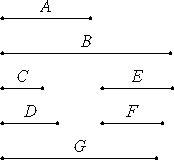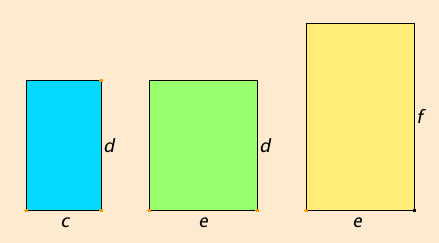# Proposition 18

Between two similar plane numbers there is one mean proportional number, and the plane number has to the plane number the ratio duplicate of that which the corresponding side has to the corresponding side.

Let A and B be two similar plane numbers, and let the numbers C and D be the sides of A, and E and F of B.

Now, since similar plane numbers are those which have their sides proportional, therefore C is to D as E is to F.

VII.Def.21

I say then that between A and B there is one mean proportional number, and A has to B the ratio duplicate of that which C has to E, or as D has to F, that is, of that which the corresponding side has to the corresponding side.VII.13

Now since C is to D as E is to F, therefore, alternately C is to E as D is to F.

And, since A is plane, and C and D are its sides, therefore D multiplied by C makes A. For the same reason also E multiplied by F makes B.

VII.17

Multiply D by E to make G. Then, since D multiplied by C makes A, and multiplied by E makes G, therefore C is to E as A is to G.

VII.17

But C is to E as D is to F, therefore D is to F as A is to G. Again, since E multiplied by D makes G, and multiplied by F makes B, therefore D is to F as G is to B.

But it was also proved that D is to F as A is to G, therefore A is to G as G is to B. Therefore A, G, and B are in continued proportion.

Therefore between A and B there is one mean proportional number.

I say next that A also has to B the ratio duplicate of that which the corresponding side has to the corresponding side, that is, of that which C has to E or D has to F.

V.Def.9

Since A, G, and B are in continued proportion, A has to B the ratio duplicate of that which it has to G. And A is to G as C is to E, and as D is to F. Therefore A also has to B the ratio duplicate of that which C has to E or D has to F.

Therefore, between two similar plane numbers there is one mean proportional number, and the plane number has to the plane number the ratio duplicate of that which the corresponding side has to the corresponding side.

Q.E.D.

## Guide

This proposition generalizes VIII.11 from squares to similar rectangles.

#### Outline of the proof

Assume that the similar plane numbers are cd and ef so that c : d = e : f, or, alternately, c : e = d : f. Then c : e = cd : de, and d : f = de : ef, therefore the ratio of the plane numbers cd : ef is compounded of the ratios of the corresponding sides c : e and d : f. Also, since the ratios of the corresponding sides are the same, the ratio of the plane numbers is the duplicate of the ratio of the sides. Furthermore, since cd : de = de : ef, the number de is a mean proportional between the two plane numbers cd and ef.#### Use of this proposition

This proposition is used in several of the remaining propositions in this book beginning with the next. It is also used in the first two propositions of Book IX. Proposition VIII.20 is a partial converse of this one.